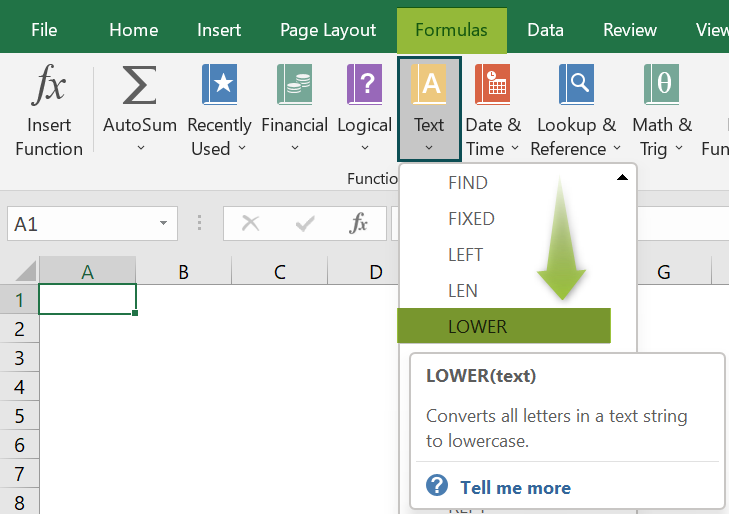# LOWER Excel FunctionArticle byExcelMojo Team## What Is LOWER Function In Excel?

The LOWER Excel function converts the uppercase or capital letters of the selected string into lowercase or small letters. LOWER function is an in-built Text function, so we can insert the formula from the “Function Library” or enter it directly in the worksheet. For example, we will use the LOWER function to return the lowercase string.

Select cell B2, enter the formula =LOWER(A2), and press the “Enter” key. The result is the lowercase “a”, as shown below. Therefore, the capital letter is converted to a small letter.

###### Key Takeaways
• The LOWER Excel function converts all the capital letters in the selected text into lowercase letters.
• In a combination of uppercase and lowercase letters like ABCDefgh, the lowercase letters remain untouched, and the function will convert to lowercase and give the result as abcdefgh.
• The function reduces the time consumption in rewriting and recorrecting the strings in a large dataset.
• We can generate email ids from names using this function by using the “&” symbol to merge the names with the domain name.

### LOWER() Excel Formula

The syntax of the LOWER Excel formula is,

The argument of the LOWER Excel formula is,

• text: It is a mandatory argument. It is only the text string that will be converted to lowercase.

### How To Use LOWER Excel Function?

We can use the LOWER Excel function in 2 ways, namely,

1. Access from the Excel ribbon.
2. Enter in the worksheet manually.

#### Method #1 – Access from the Excel ribbon

Choose an empty cell for the output → select the “Formulas” tab → go to the “Function Library” group → click the “Text” option drop-down → select the “LOWER” function, as shown below.

The “Function Arguments” window appears. Enter the argument in the “text” field → click “OK”, as shown below.

#### Method #2 – Enter in the worksheet manually

1. Select an empty cell for the output.
2. Type =LOWER( in the selected cell. [Alternatively, type =L or =LO and double-click the LOWER function from the list of suggestions shown by Excel.]
3. Enter the arguments as cell values or cell references in Excel and close the brackets.
4. Press the “Enter” key.

Let us take a basic example to understand its application.

We will convert the uppercase fruit names to lowercase using LOWER Excel function.

In the table, the data is,

• Column A contains the Value.
• Column B contains the Output.

The steps to change the case using LOWER formula are,

1. Select cell B2, enter the formula =LOWER(A2), and press the “Enter” key. The result is “apple”.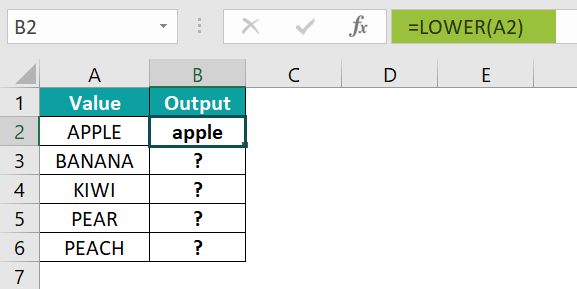2. Drag the formula from cell B2 to B6 using the fill handle. The output is shown below.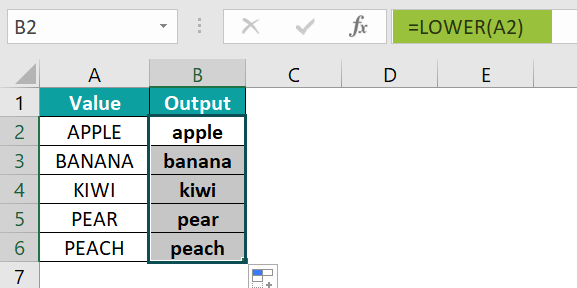### Examples

We will understand some advanced scenarios using the LOWER Excel function examples.

#### Example #1

We will convert the case using the LOWER function for the month’s name of the year.

In the table, the data is,

• Column A contains the Value.
• Column B contains the Output.

The steps to change the case using the LOWER formula are,

• Step 1: Select cell B2, enter the formula =LOWER(A2), and press the “Enter” key. The result is “january”.
• Step 2: Drag the formula from cell B2 to B13 using the fill handle. The output is shown below.

#### Example #2

We will convert the case using the LOWER function for the names in all uppercase letters.

In the table, the data is,

• Column A contains the Name.
• Column B contains the Output.

The steps to change the case using the LOWER formula are,

• Step 1: Select cell B2, enter the formula =LOWER(A2), and press the “Enter” key. The result is “ron”.
• Step 2: Drag the formula from cell B2 to B5 using the fill handle. The output is shown below.

#### Example #3

We will form the email by converting the case using the LOWER function for the first and last names.

In the table, the data is,

• Column A contains the First Name.
• Column B contains the Last Name.
• Column C contains the Email.

The steps to change the case using the LOWER formula are,

• Step 1: Select cell C2, enter the formula =LOWER(A2)&LOWER(B2)&“@xyz.com”, and press the “Enter” key. The result is “johnpeter@xyz.com”.
• Step 2: Drag the formula from cell C2 to C5 using the fill handle. The output is shown below.

### Important Things To Note

• Lower Excel function does not change the punctuation characters, symbols, etc., it returns as it is.
• If there is a space between words, or the cell value is a numeric value, the function does not consider that.
• If the cell values or reference is blank, then the result after applying the formula will be blank.

1. What is the LOWER Excel function used for?

The LOWER Excel function converts each character in a selected string into lowercase.

The formula of the LOWER function is =LOWER(text)

For example, we will convert all uppercase letters into lowercase using the LOWER form

In the table, the data is,
Column A contains the Value.
Column B contains the Output.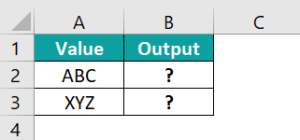The procedure to change the case using the LOWER formula is,

Select cell B2, enter the formula =LOWER(A2), press the “Enter” key, and drag the formula from cell B2 to B3 using the fill handle.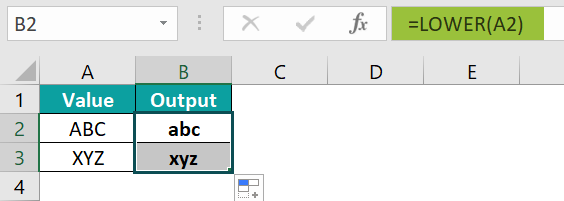We will get the output shown above.

2. How to insert the LOWER Excel function?

We can insert the LOWER function as follows:

1. Select an empty cell for the output.
2. Type =LOWER( in the selected cell. [Alternatively, type =L or =LO and double-click the LOWER function from the list of suggestions shown by Excel.]
3. Enter the arguments as cell values or cell references and close the brackets.
4. Press the “Enter” key.

For example, we will convert all uppercase letters into lowercase using the LOWER Function.

In the table, the data is,
Column A contains the Value.
Column B contains the Output.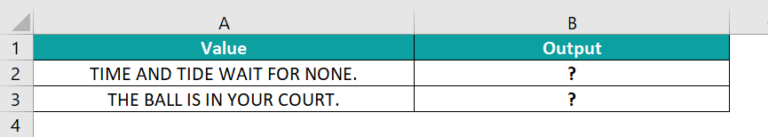The procedure to change the case using the LOWER formula is,

Select cell B2, enter the formula =LOWER(A2), press the “Enter” key, and drag the formula from cell B2 to B3 using the fill handle.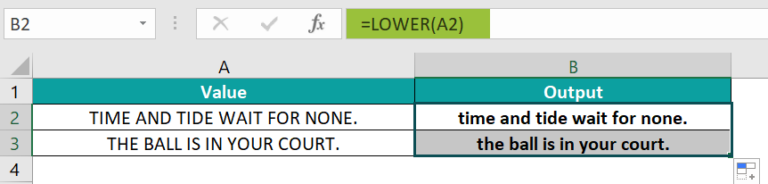We will get the output shown above in column B.

3. Where is the LOWER Excel function?

The LOWER function is in the “Formulas” tab and can be used as follows:

Choose an empty cell for the output → select the “Formulas” tab → go to the “Function Library” group → click the “Text” option drop-down → select the “LOWER” function, as shown below.